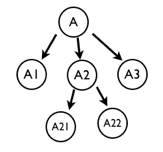Light High Efficiency Graph Component

# Traverse by Tree Graph

lements in graph model form a tree according to set membership. Traverses by tree map. Two methods are provided, separately depth first and breath first. The depth first traversal is divided into the preorder traversal and the postorder traversal. In addition, the reverse transversal is supported

• #forEachByDepthFirst(call, scope, postOrder) - The depth first traversal is divided into the preorder traversal and the postorder traversal
• #forEachByDepthFirstReverse(call, scope, postOrder) - The depth first reverse traversal is divided into the preorder traversal and the postorder traversal

## Set membership

The set membership is showed in the following graph. The arrow indicates the set relation. Different call sequences will be realized by different traversal methodExample

``````var model = new Q.GraphModel();
var a = new Q.Node('A');
var a1 = new Q.Node('A1');
var a2 = new Q.Node('A2');
var a3 = new Q.Node('A3');
var a21 = new Q.Node('A21');
var a22 = new Q.Node('A22');
a1.parent = a;
a2.parent = a;
a21.parent = a2;
a22.parent = a2;

Q.log(node.name);
}, null, true);

Q.log('forEachByDepthFirst by post-order');
model.forEachByDepthFirst(function(node){
Q.log(node.name);
}, null, true);

Q.log('forEachByDepthFirst by pre-order');
model.forEachByDepthFirst(function(node){
Q.log(node.name);
});
``````

The traversal results are showed as follows:

``````A

A3

A1

A2

A21

A22
``````

forEachByDepthFirst by post-order

``````A1

A21

A22

A2

A

A3
``````

forEachByDepthFirst by pre-order

``````A

A1

A2

A21

A22

A3
``````